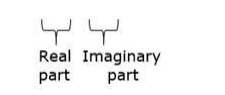# Simplify each of the following and express it in the form a + ib :

Question:

Simplify each of the following and express it in the form a + ib :

$(-5+6 i)-(-2+i)$

Solution:

Given: $(-5+6 i)-(-2+i)$

Firstly, we open the brackets

$-5+6 i+2-i$

$=-3+5 i$